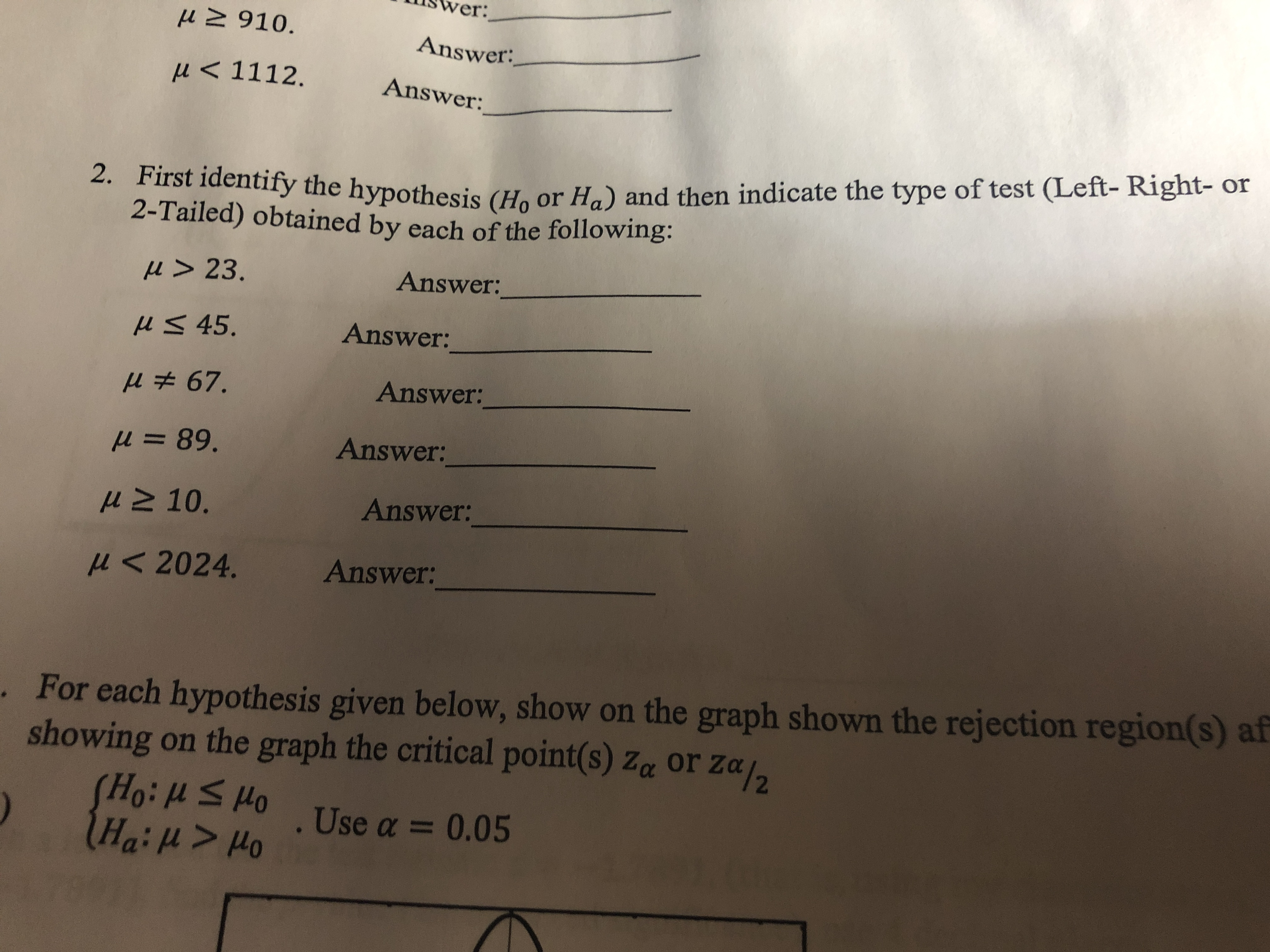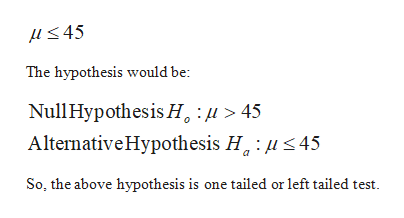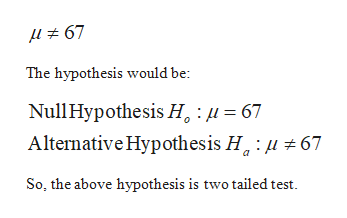# Swer:Answer:P 1112.Answer:2. First identify the hypothesis (Ho or Ha) and then indicate the type of test (Left- Right- or2-Tailed) obtained by each of the following:u> 23.Answer:uS 45.Answer:Answer:89.Answer:uM 10.Answer:2024.Answer:For each hypothesis given below, show on the graph shown the rejection region(s) afshowing on the graph the critical point(s) z or ZaOod 5 1:0H).Use a 0.05Ha:u> Ho

Question
1 viewshelp_outlineImage TranscriptioncloseSwer: Answer: P 1112. Answer: 2. First identify the hypothesis (Ho or Ha) and then indicate the type of test (Left- Right- or 2-Tailed) obtained by each of the following: u> 23. Answer: uS 45. Answer: Answer: 89. Answer: uM 10. Answer: 2024. Answer: For each hypothesis given below, show on the graph shown the rejection region(s) af showing on the graph the critical point(s) z or Za Ood 5 1:0H) .Use a 0.05 Ha:u> Ho fullscreen
check_circle

Step 1

Given:

Step 2

Given:help_outlineImage TranscriptioncloseL 45 The hypothesis would be Null Hypothesis H, 45 Alternative Hypothesis H 45 So, the above hypothesis is one tailed or left tailed test fullscreen
Step 3

Given:

...help_outlineImage Transcriptionclose67 The hypothesis would be Null Hypothe sis H, 67 Alternative Hypothesis H 67 So, the above hypothesis is two tailed t fullscreen

### Want to see the full answer?

See Solution

#### Want to see this answer and more?

Solutions are written by subject experts who are available 24/7. Questions are typically answered within 1 hour.*

See Solution
*Response times may vary by subject and question.
Tagged in

### Hypothesis Testing